# PSAT Math : How to find out if a number is prime

## Example Questions

### Example Question #1 : Prime Numbers

The sum of the first seven prime numbers divided by two is

33

61

58

24

29

29

Explanation:

The first seven primes are 2, 3, 5, 7, 11, 13, and 17. Don't forget about 2, the smallest prime number, and also the only even prime! Adding these seven numbers gives a sum of 58, and 58/2 = 29.

### Example Question #18 : How To Find Out If A Number Is Prime

If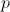is a prime number, what could also be prime?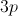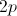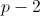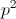Explanation:

Plug in a prime number such as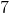and evaluate all the possible solutions. Note that the question asks which value COULD be prime, not which MUST BE prime. As soon as your number-picking yields a prime number, you have satisfied the "could be prime" standard and know that you have a correct answer.

### Example Question #2 : Prime Numbers

How many integers between 2 and 20, even only, can be the sum of two different prime numbers?Explanation:

There are 8 possible numbers; 4,6,8,10,12,14,16,18.

One is not a prime number, so only 8, 10, 12, 14, 16, and 18 can be the sum of two different prime numbers.

### Example Question #3 : Prime Numbers

Define a series of consecutive prime numbers to be a series of numbers, each prime, in which there are no other prime numbers between them.  These are not necessarily consecutive numbers themselves.  For example, the numbers 5,7 and 11 are consecutive prime numbers, although they are not consecutive numbers.

Ifis the first number in a series ofprime numbers, which of the following could not be the value of the last number in the series?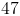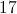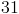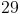Explanation:

The primes, in order, are:

2, 3, 5, 7, 11, 13, 17, 19, 23, 29, 31, 37, 41, 43, 47, 53, ...

We create a few series:-> series length 2: 2,3-> series length 3: 3,5,7-> series length 5: 5,7,11,13,17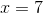-> series length 7: 7,11,13,17,19,23,29

etc.

We can then see that, of the answers, only 47 and 31 remain possibly correct answers.  Now we need to decide which of those two are impossible.

We could do another series, but the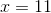series has 11 terms requiring us to go further and further up.  If we do this, we'll find that it terminates at 47, meaning that 31 must be the correct answer.

Another way, however, is to notice that 29 is the end of theseries.  Since 31 is the very next prime number, if we start on 11, the series that terminates in 31 would have to have length 7 as well.  Every series afterwill thus end on a number larger than 31, meaning we will never finish on a 31.

### Example Question #4 : Prime Numbers

Ifis a prime number, how many factors does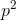have?Explanation:

The value of, or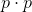, is the product ofand, so it will be divisible by 1, p, p, and nothing else (we know that the p’s are not divisible because they are prime). Therefore p2 has exactly three factors.

(Alternatively, we can plug in any prime number for p and see how many factors p2 has. For example, if p is 3, then the factors of p2, or 9, are 1, 3, and 9.)

### Example Question #1 : How To Find Out If A Number Is Prime

The sum of four consecutive integers is 210.  Which one of these four integers is prime?

51

49

47

57

53

53

Explanation:

Let x represent the smallest of the four numbers.

Then we can set up the following equation: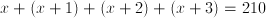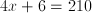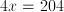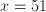Therefore the four numbers are 51, 52, 53, 54.  The only prime in this list is 53.

### Example Question #1 : How To Find Out If A Number Is Prime

Which of the following is equal to the sum of the five smallest prime numbers?

25

27

33

18

28

28

Explanation:

It is important to know what a prime number is in order to answer this question. A prime number is defined as any positive integer that is divisible only by the number 1 and itself. For example, 17 is a prime number because its only factors are 1 and 17.

The first five prime numbers are 2, 3, 5, 7, and 11. Remember, 1 is not a prime number, and 2 is the only even prime.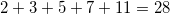Therefore, the sum of the five smallest prime numbers is 28.